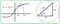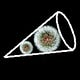An infinitesimal portion of the curve, S looks like a straight line, dS.

# The Length of a Curve

## The arc length formula using infinitesimals

wish to derive a general formula for the arc length of a curve given by the function, y=f(x). We will do so using infinitesimals. These are infinitely small portions of the curve.

The figure below shows such a curve. The length of the segment between f(x₀) and f(x₁) is S. The circle to the right shows an infinitely small portion of the segment, dS. At such a close range, dS is a straight line segment. It forms the hypotenuse of a right triangle with legs, dS and dy. The angle between dS and dx is dθ.An infinitesimal portion of the curve, S looks like a straight line, dS.

The ratio of dS to dx is sec dθ.

We can move dx to the right-hand side of the expression. Once we integrate between x₀ and x₁, we have an expression for S.

We have a problem. The variable of integration is θ, whereas the differential is in terms of x. This won’t do. We begin by translating the integrand into a more useful form.

This form is more useful to us. We can take tan² θ and express it in terms of dx is dy.

We now have our general formula for the length of the segment, S.

Written by

Written by

#### Adam Hrankowski is a maths/physics tutor in BC. His book, "When Am I Ever Gonna Use This Stuff” is available for preorder at: https://amzn.to/3egZUib.